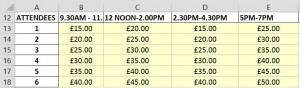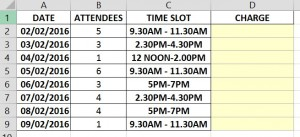# INDEX and MATCH Functions

These functions give you greater flexibility then the Vlookup or Hlookup functions because they allow you to do a two-way (Matrix) lookup. Therefore, you can search for two lookup values in one table (data).

The table lists the number of Attendees as the Vertical lookup and the Time Slots as the Horizontal Lookup.Note: A matrix lookup will only work if your table containing the data has values at the top (row headings) and on the left hand side (column headings).

In the example below I am managing the bookings for a meeting room. The meeting room has various charges according to the number of attendees and the time slot, they wish to book, and I want to populate the cells D2:D9 with the correct charge contained in my table in A12:E18 above.To perform this we use the INDEX and MATCH functions. The syntax for this is:

=INDEX(array, MATCH( lookup-value, lookup-array, 0), MATCH(horizontal lookup value, horizontal lookup row,0))

Now, how to use these functions in relation to the information we have above.

1. Click in cell D2
2. Type =INDEX(\$A\$12:\$E\$18,
3. This locates the Table Array with the various prices for the room rental.

Note: We are using absolute referencing for the data in the table_array as this is always going to be in the same place. However, for the Attendees and Time Slots cells when we copy the formula down from D2 we will want their references to be relative.

1. Now type MATCH(B2,\$A\$13:\$A\$18,0)

B2 is the number of attendees and A13:A18 are the different attendee numbers in the table_array. The Vlookup values.

1. Now type MATCH(C2,\$B\$12:\$E\$12,0))
2. C2 is the time slot and B12:E12 are the different time slots intervals in the table_array. The Hlookup values.
3. Copy the formula down from D2 to D9 to collect the correct rate for the correct number of Attendee

Note: The Zero (0) means that it is an exact match.